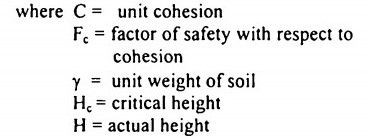top of pageSearch

# Soil Mechanics (121-140)

Updated: Apr 27, 2020

121. Sensitivity of a soil can be defined as ratio of compressive strength of

unconfined undisturbed soil to that of soil in a remoulded state

122. Rankine's theory of earth pressure assumes that the back of the wall is

vertical and smooth

123. The coefficient of active earth pressure for a loose sand having an angle of internal friction of 30° is 1/3

124. The major principal stress in an element of cohesionless soil within the backfill of a vertical if the soil is in an active state of plastic equilibrium

125. Passive earth pressure in a soil mass is proportional towhere μ is Poisson's ratio and Φ is the effective angle of internal friction of soil

126. The effect of cohesion on a soil is to reduce the active earth pressure in

tensity but to increase the passive earth pressure intensity

127. A retaining wall 6m high supports a backfill with a surcharge angle of 10°. The

back of the wall is inclined to the vertical at a positive batter angle of 5°. If the angle of wall friction is 7°, then the resultant active earth pressure will act at a distance of 2 m above the base and inclined to the horizontal at an angle of

12°

128. Coefficient of earth pressure at rest is greater than active earth pressure but

less than passive earth pressure

129. If the top surface of the backfill of a retaining wall is inclined to the horizontal

at an angle β, then the coefficient of passive earth pressure is equal to130. The critical height of an unsupported vertical cut in a cohesive soil is given by131. Total lateral earth pressure is proportional to square of depth of soil

132.Cohesive soils are poor for backfill because of large lateral pressure

133.Which of the following earth pressure theories is directly applicable to bulk

134. Taylor's stability number is equal to135. For a base failure, the depth factor Df is D f> 1

136.Base failure of a finite slope occurs when the soil below the toe is relatively soft and weak

137.Bishop's method of stability analysis assumes the slip surface as an arc of a

circle

138.Allowable bearing pressure for a foundation depends upon both allowable settlement and ultimate bearing capacity

139.According to Rankine's analysis, minimum depth of foundation is equal toγ = unit weight of soil

Φ = angle of internal friction

140.According to Terzaghi's theory, the. ultimate bearing capacity at ground surface for a purely cohesive soil and for a smooth base of a strip footing is

5.14 C